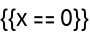#Function Repository Resource:

# FunctionDiscontinuities

Compute the discontinuities of a function of a single variable

Contributed by: Wolfram|Alpha Math Team
 ResourceFunction["FunctionDiscontinuities"][f,x] computes the values of x at which f(x) is discontinuous with respect to x. ResourceFunction["FunctionDiscontinuities"][{f,cond},x] computes the x for which cond is True and f(x) is discontinuous with respect to x. ResourceFunction["FunctionDiscontinuities"][…,x,"Properties"] computes points of discontinuity along with related information about each.

## Details and Options

ResourceFunction["FunctionDiscontinuities"] has the attribute HoldFirst.
ResourceFunction["FunctionDiscontinuities"] takes the option "ExcludeRemovableSingularities", having default value False, that determines whether to exclude removable discontinuities from the result. A function f(x) is said to have a removable discontinuity at a point x=a if the limit of f(x) as xa exists and is independent of the direction in which the limit is taken, but has a value that is different from f(a) (which may or may not be defined).

## Examples

### Basic Examples

Compute the points of discontinuity of a rational function:

 In:=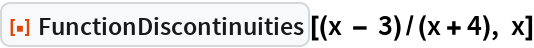Out=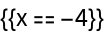Repeat the calculation, classifying the points of discontinuity:

 In:=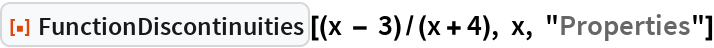Out=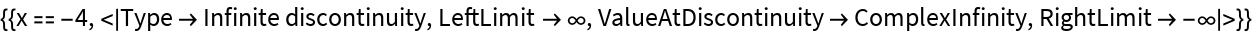Compute the points of discontinuity of a trigonometric function:

 In:=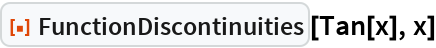Out=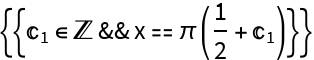Repeat the calculation, classifying the points of discontinuity:

 In:=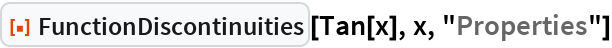Out=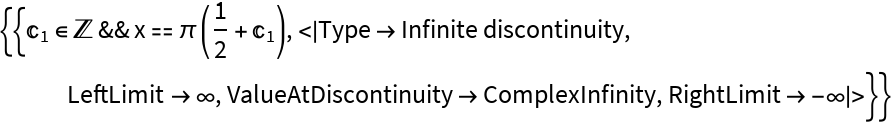Compute the points of discontinuity of a smooth function:

 In:=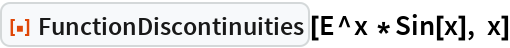Out=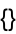Compute and plot the points of discontinuity of a rational function:

 In:=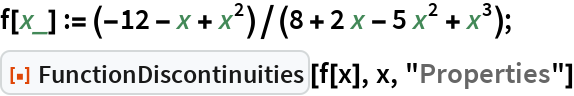Out=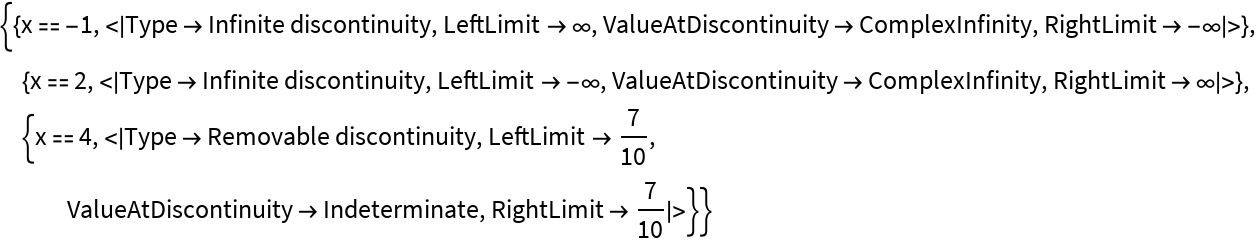In:=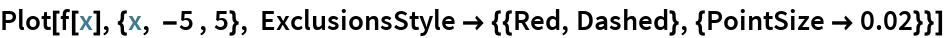Out=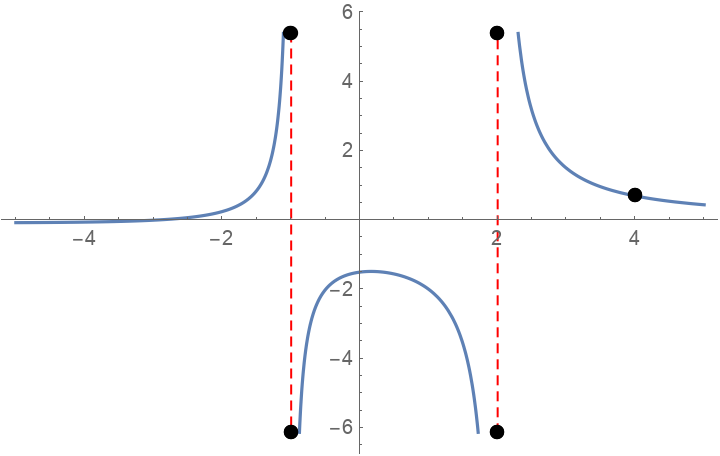Compute and plot the points of discontinuity of a step function:

 In:=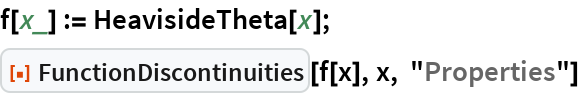Out=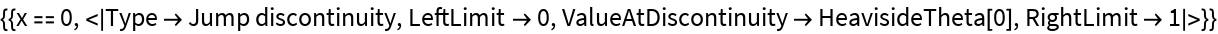In:=Out=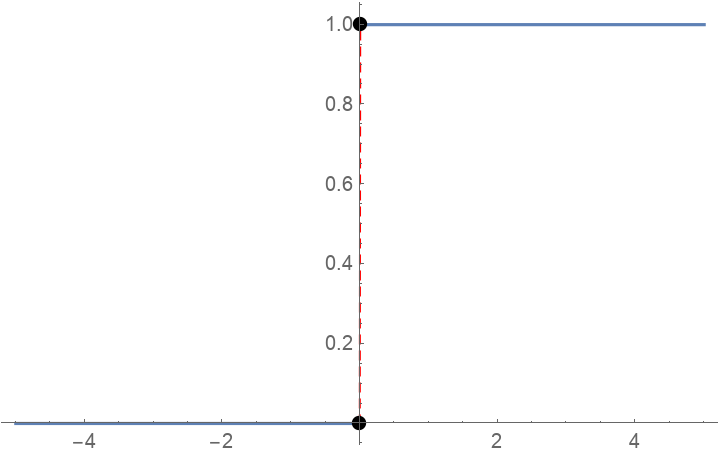Compute and plot the points of discontinuity of a step function:

 In:=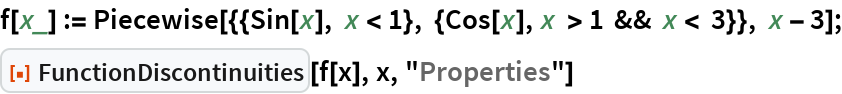Out=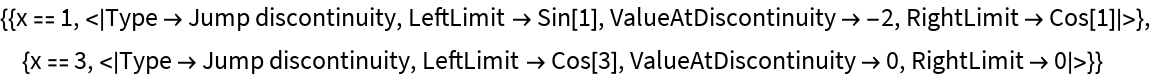In:=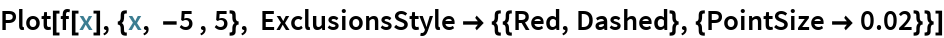Out=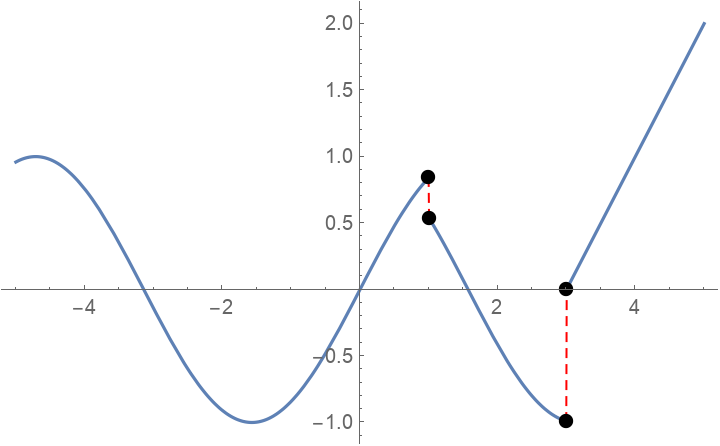### Options

Choose whether to exclude removable singularities:

 In:=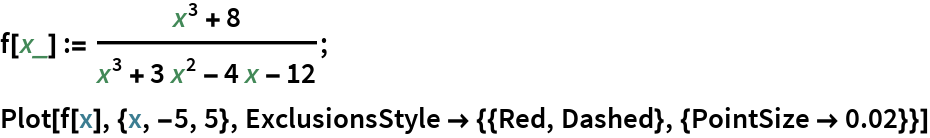Out=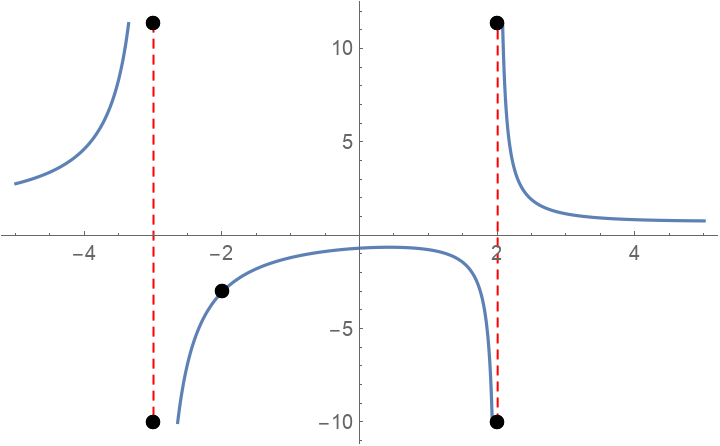In:=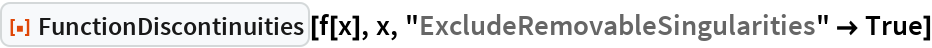Out=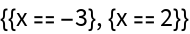In:=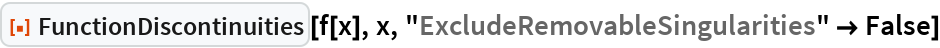Out=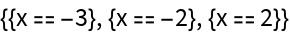### Properties and Relations

FunctionDiscontinuities has the attribute HoldFirst, enabling calculations such as the following:

 In:=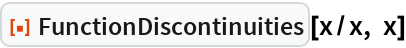Out=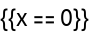### Possible Issues

Points where a function approaches ±∞ are considered to be points of discontinuity, even if they are technically outside the range of function definition:

 In:=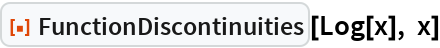Out=